A Taste of

#### Topological Data Analysis (TDA)

##### (Shape Reconstruction)
Dr. Sushovan Majhi
School of Information, UCB
January 13, 2022Slides: smajhi.com/22-sp-icfai

#### Collaborators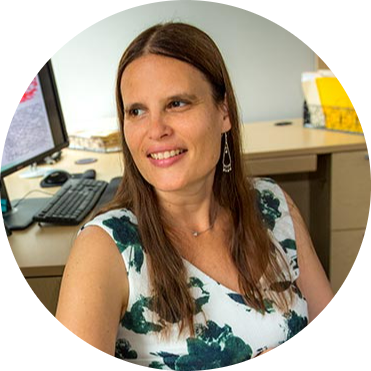Carola Wenk
Tulane University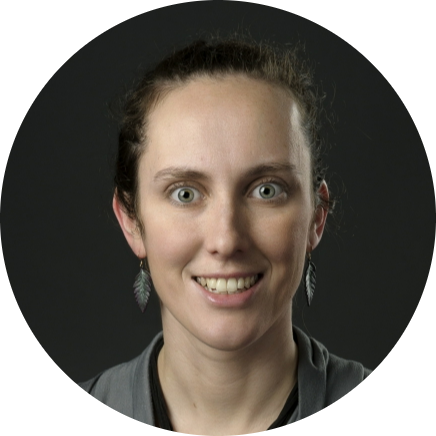Brittany Fasy
Montana State UJeff Vitter,
U of MississippiMd. Nurujjaman
National Institute of Technology
India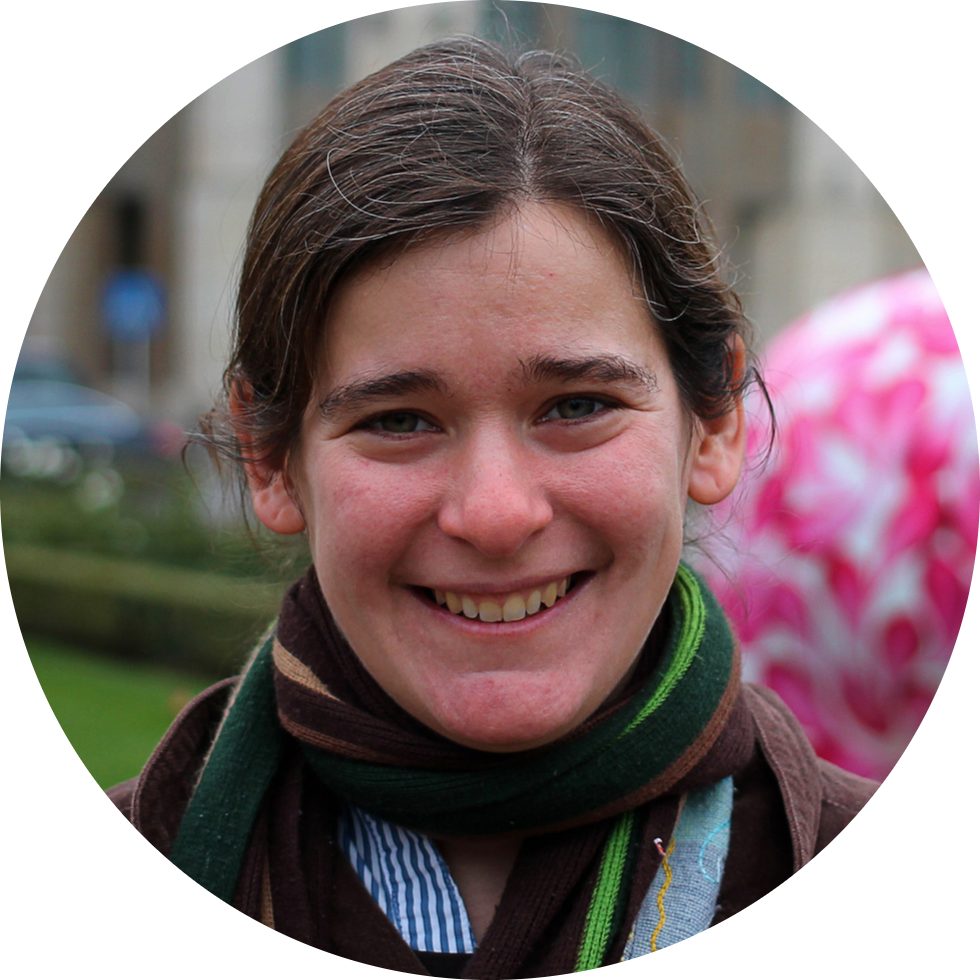Erin Chambers
St. Louis UniversityLiz Munch
Michigan State U

#### The Manifesto

• Introduction to TDA
• The Reconstruction Problem
• Vietoris-Rips Complex
• A Possible Solution
• Opportunities in TDA
• Questions

#### Topological Data Analysis (TDA)• Computational Topology
> algorithmcally compute various topological invariants, e.g., homology groups, Betti numbers, Euler characteristic, etc
• Applied Topology
> apply topological ideas in sensor network, motion planning in robotics, configuration spaces etc
• TDA
> learning from big data, (stratified) manifold learning, shape reconstruction and comparison, and so much more...

### Shape Reconstruction

#### ShapesSmooth Manifolds (the good ones)

Our shapes of interest (the ugly ones)

#### Geodesic SpacesFor any two points $x,y\in X\subseteq\mathbb{R}^N$, there exists a geodesic or shortest path.
For a geodesic space $X\subseteq\mathbb{R}^N$, the distortion $$\delta=\sup_{x\neq y}\frac{\color{red}{d_L(x,y)}}{\color{blue}{\|x-y\|}}.$$
For a geodesic space $X\subseteq\mathbb{R}^N$, the convexity radius $\rho$ is the half of the (ant) distance between a pair of furthest points $x,y\in X$ that have a unique geodesic. For a unit length circle, its convexity radius is one quarter.

#### The Reconstruction ProblemNoisy Sample (www.smajhi.com/shape-reconstruction)Sample from $S^1\vee S^1$ (www.smajhi.com/shape-reconstruction)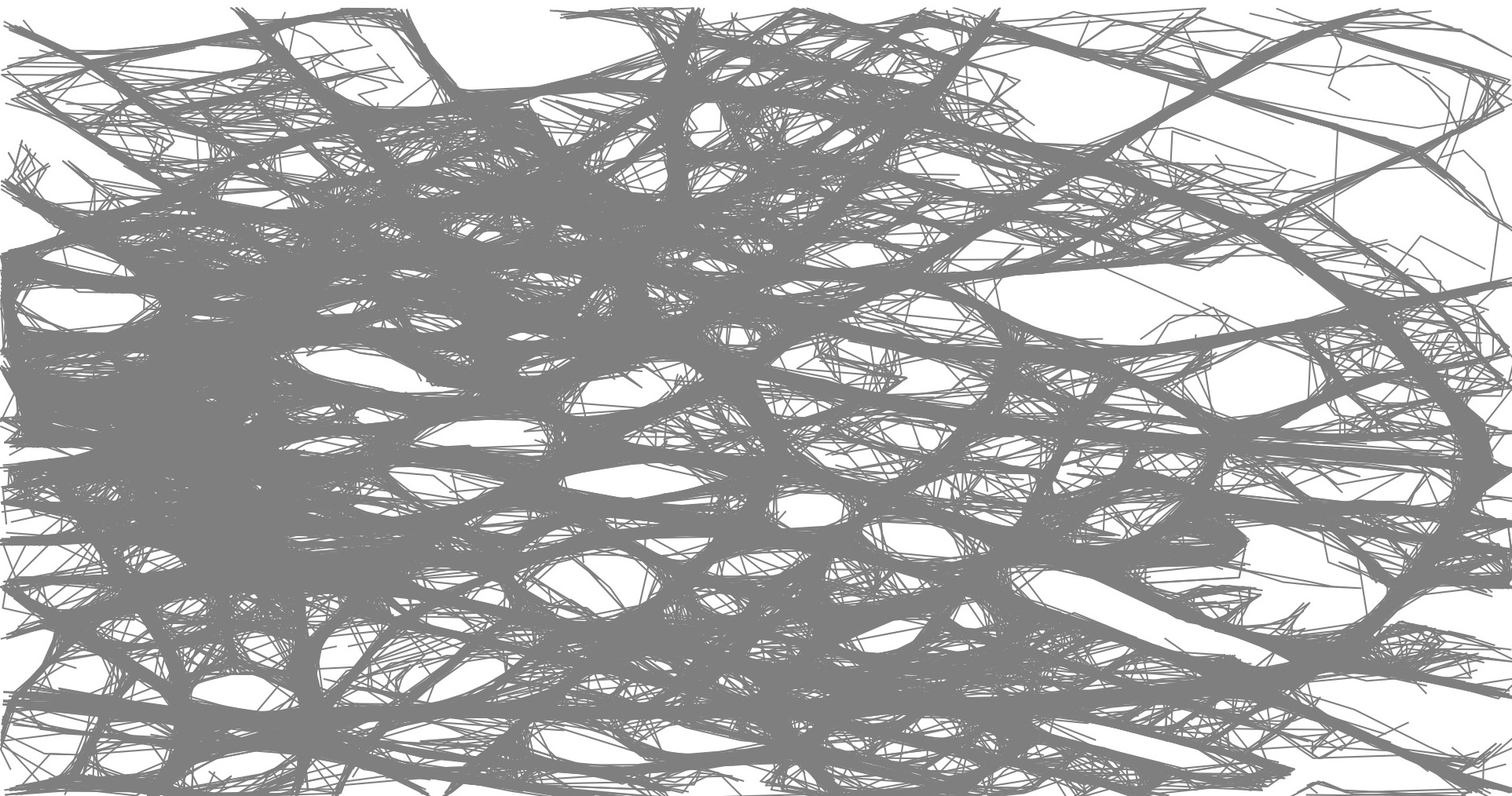GPS Traces Berlin (www.mapconstruction.org)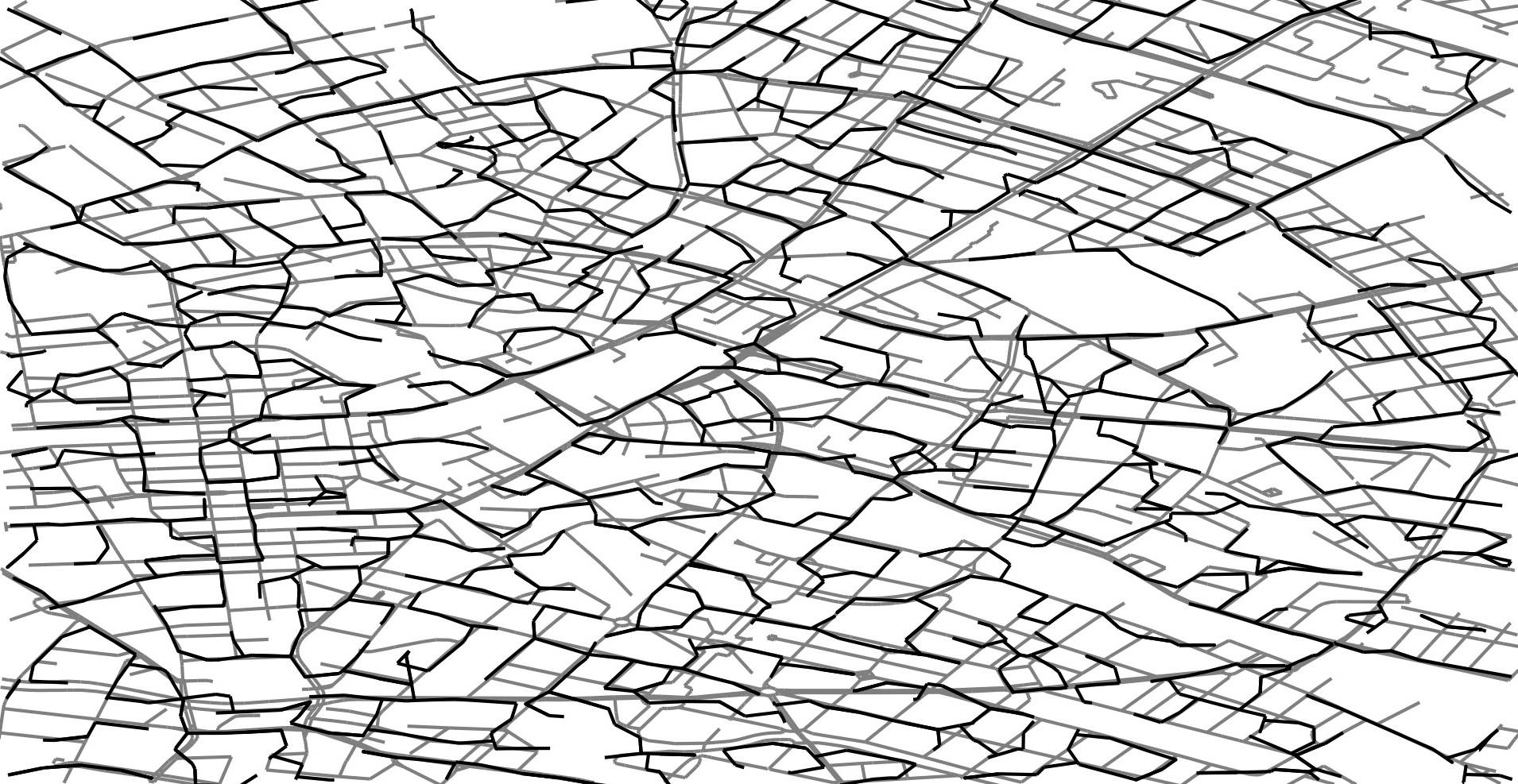#### Problem Statement

• Let $X$ be a hidden geodesic subset of $\mathbb{R}^N$
• We have a sample or a point-cloud $(S,\|\cdot\|)$
• $S$ is reasonably dense around $X$.
Q: Can we infer the toplogy of $X$ from $S$? What does inference even mean in such a reconstruction context?
• Compute $H_*(X)$ and/or $\pi_*(X,b)$ from $S$
• Construct $\widetilde{X}$ homotopy equivalent to $X$
• $\widetilde{X}$ has a geometrically-close embedding in $\mathbb{R}^N$

#### Density via Hausdorff DistanceHausdorff distance $d_H(A,B)$ is
$\max\{$ $a$, $b$ $\}=$ $b$
💡

If $d_H(A,B) <\varepsilon$, then
> each point $a\in A$ has a point $b\in B$ with $||a-b|| <\varepsilon$, and
> each point $b\in B$ has a point $a\in A$ with $||a-b|| <\varepsilon$.

#### Vietoris-Rips ComplexFor a metric space $(M,d_M)$ and scale $\varepsilon>0$, each subset $A$ of size $k$ with $diam_d(A)\leq\epsilon$ is a $(k-1)$-simplex of the Vietoris-Rips complex $VR_\varepsilon(M)$.
Let $X$ be a smooth sub-manifold. If $\epsilon>0$ is small and $d_H(X,S)$ is also small, then the Vietoris-Rips complex $VR_\varepsilon(S)$ is homotopy equivalent to $X$.

#### Geodesic Spaces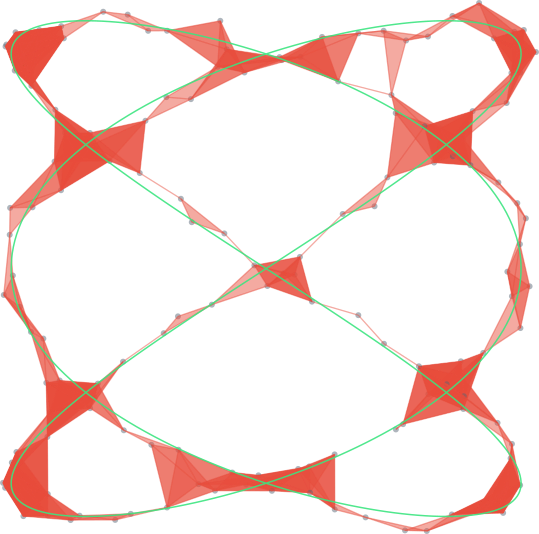small $\epsilon$ + dense sample trick
does not work
😢

#### Our Approach

• $X\subseteq\mathbb{R}^N$ is a geodesic subspace $(X,d_L)$
• Distortion $\delta$ is finite
• Convexity radius $\rho$ is positive
• $d_H(X,S)$ is small

#### Topological Reconstruction

If $d_H(X,S) <\epsilon<\frac{\rho}{2\delta(3\delta+1)}$, the image $$Im(j_*)\simeq\pi_*(X),$$ where $j:\mathcal{VR}_{\epsilon}(S)\to\mathcal{R}_{\frac{1}{2}(3\delta+1)\epsilon}(S)$.

#### Geometric Reconstruction

If $d_H(G,S) <\epsilon<\frac{\rho}{2\delta(3\delta+1)}$, we can output $\widetilde{X}$ such that $$\widetilde{X}\simeq G,$$ and $d_H(G,\widetilde{X})$ is small.

#### Remarks on Reconstruction

• Our geometric reconstruction works for planar graphs. Spatial graphs? My very recent work!
• Geometric reconstruction of higher-dimensional objects, like embedded simplicial complexes.
• Incorporate statistical treatments.
• The visualization tool is available as a webapp (github.com/sushovan4/shape-reconstruction). Need volunteers to extend it.

#### Opportunities

• Companies like Ayasdi are hiring topological data scientists and interns
• Open-source projects in TDA: Gudhi (C++), Scikit-tda (Python), tdajs (Typescript/JS)
• Graduate schools like OSU, Michigan S, Duke, Montana State, Tulane have TDA grants/projects
• I also have some cool problems and projects (theory and application)
smajhi@berkeley.edu